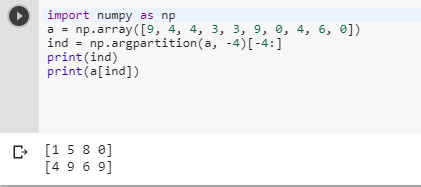2 views
in Python

NumPy proposes a way to get the index of the maximum value of an array via np.argmax.

I would like a similar thing, but returning the indexes of the N maximum values.

For instance, if I have an array, [1, 3, 2, 4, 5], function(array, n=3) would return the indices [4, 3, 1] which correspond to the elements [5, 4, 3].

by (106k points)

For getting the indices of N maximum values in a NumPy array we have Newer NumPy versions (1.8 and up) that have a function called argpartition. To get the indices of the four largest elements, do

>>> a = np.array([9, 4, 4, 3, 3, 9, 0, 4, 6, 0])

>>> ind = np.argpartition(a, -4)[-4:]

>>> ind

>>> a[ind]+1 vote